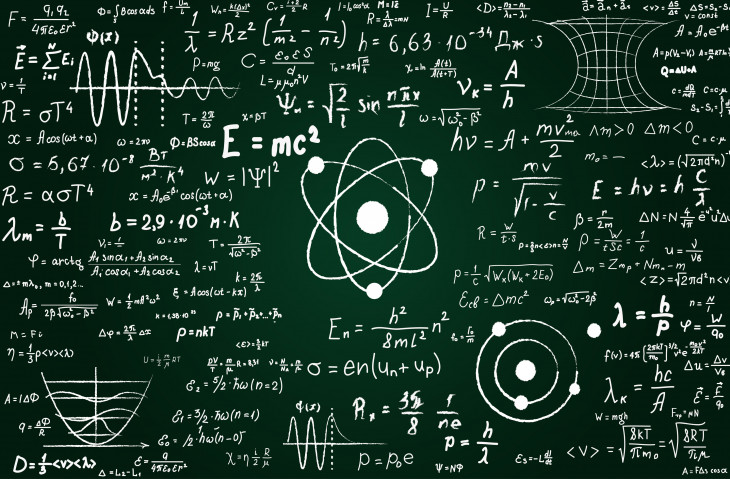# Mathematicians - What They DoMathematics is one of the oldest and most fundamental sciences. Mathematicians use mathematical theory, computational techniques, algorithms, and the latest computer technology to solve economic, scientific, engineering, and business problems. The work of mathematicians falls into two broad classes: theoretical (pure) mathematics and applied mathematics. These classes, however, are not sharply defined and often overlap.

Theoretical mathematicians advance mathematical knowledge by developing new principles and recognizing previously unknown relationships between existing principles of mathematics. Although these workers seek to increase basic knowledge without necessarily considering its practical use, such pure and abstract knowledge has been instrumental in producing or furthering many scientific and engineering achievements. Many theoretical mathematicians are employed as university faculty, dividing their time between teaching and conducting research.

Applied mathematicians use theories and techniques, such as mathematical modeling and computational methods, to formulate and solve practical problems in business, government, engineering, and the physical, life, and social sciences. For example, they may analyze the most efficient way to schedule airline routes between cities, the effects and safety of new drugs, the aerodynamic characteristics of an experimental automobile, or the cost-effectiveness of alternative manufacturing processes.

Applied mathematicians working in industrial research and development may develop or enhance mathematical methods when solving a difficult problem. Some mathematicians, called cryptanalysts, analyze and decipher encryption systems—codes—designed to transmit military, political, financial, or law-enforcement-related information.

Applied mathematicians start with a practical problem, envision its separate elements, and then reduce the elements to mathematical variables. They often use computers to analyze relationships among the variables, and they solve complex problems by developing models with alternative solutions.

Individuals with titles other than mathematician also do work in applied mathematics. In fact, because mathematics is the foundation on which so many other academic disciplines are built, the number of workers using mathematical techniques is much greater than the number formally called mathematicians. For example, engineers, computer scientists, physicists, and economists are among those who use mathematics extensively. Some professionals, including statisticians, actuaries, and operations research analysts, are actually specialists in a particular branch of mathematics. Applied mathematicians frequently are required to collaborate with other workers in their organizations to find common solutions to problems.

Work Environment

Mathematicians held about 2,700 jobs in 2020. The largest employers of mathematicians were as follows:

• Federal government - 47%
• Professional, scientific, and technical services - 26%
• Colleges, universities, and professional schools; state, local, and private - 17%

Statisticians held about 42,000 jobs in 2020. The largest employers of statisticians were as follows:

• Research and development in the physical, engineering, and life sciences - 14%
• Federal government - 12%
• Healthcare and social assistance - 9%
• Insurance carriers and related activities - 8%
• Colleges, universities, and professional schools; state, local, and private 7%

Mathematicians and statisticians typically work in offices. They also may work on teams with engineers, scientists, and other specialists.

### Work Schedules

Most mathematicians and statisticians work full time. Deadlines and last-minute requests for data or analysis may require overtime. In addition, these workers may travel to attend seminars and conferences.

Education & Training Required

In private industry, candidates for mathematician jobs typically need a Ph.D., although there may be opportunities for those with a master's degree. Most of the positions designated for mathematicians are in research-and-development laboratories, as part of technical teams.

In the Federal Government, entry-level job candidates usually must have at least a bachelor's degree with a major in mathematics or 24 semester hours of mathematics courses. Outside the Federal Government, bachelor's degree holders in mathematics usually are not qualified for most jobs, and many seek advanced degrees in mathematics or a related discipline. However, bachelor's degree holders who meet State certification requirements may become primary or secondary school mathematics teachers.

Most colleges and universities offer a bachelor's degree in mathematics, and many universities offer master's and doctoral degrees in pure or applied mathematics. Courses usually required for these programs include calculus, differential equations, and linear and abstract algebra. Additional courses might include probability theory and statistics, mathematical analysis, numerical analysis, topology, discrete mathematics, and mathematical logic. In graduate programs, students also conduct research and take advanced courses, usually specializing in a subfield of mathematics.

Many colleges and universities advise or require students majoring in mathematics to take courses in a closely related field, such as computer science, engineering, life science, physical science, or economics. A double major in mathematics and another related discipline is particularly desirable to many employers. High school students who are prospective college mathematics majors should take as many mathematics courses as possible while in high school.

Other Skills Required (Other qualifications)

For jobs in applied mathematics, training in the field in which mathematics will be used is very important. Mathematics is used extensively in physics, actuarial science, statistics, engineering, and operations research. Computer science, business and industrial management, economics, finance, chemistry, geology, life sciences, and behavioral sciences are likewise dependent on applied mathematics. Mathematicians also should have substantial knowledge of computer programming, because most complex mathematical computation and much mathematical modeling are done on a computer.

Mathematicians need to have good reasoning to identify, analyze, and apply basic principles to technical problems. Communication skills also are important, because mathematicians must be able to interact and discuss proposed solutions with people who may not have extensive knowledge of mathematics.

Mathematicians - What They Do - Page 2